﻿ 一种顾及有色噪声的四星座GNSS动态导航滤波算法文章快速检索 高级检索
 大地测量与地球动力学2019, Vol. 39Issue (6): 625-629  DOI: 10.14075/j.jgg.2019.06.014引用本文SUN Qingfeng, CAI Changsheng, CUI Xianqiang, et al. A Filtering Algorithm for Quad-Constellation GNSS Kinematic Navigation with Consideration of Colored Noise[J]. Journal of Geodesy and Geodynamics, 2019, 39(6): 625-629.Foundation support

National Key Research and Development Program of China, No. 2016YFB0501803; National Natural Science Foundation of China, No. 41674039, 41674012.

About the first author

SUN Qingfeng, postgraduate, majors in GNSS navigation and positioning, E-mail: luosu_sqf@163.com.

文章历史

1. 中南大学地球科学与信息物理学院，长沙市麓山南路932号，410083

1 函数模型拟合滤波有色噪声处理算法

 $\left. \begin{array}{l} {P^{\rm{G}}} = {\rho ^{\rm{G}}} + c{\rm{d}}t - c{\rm{d}}{T^{\rm{G}}} + d_{{\rm{orb}}}^{\rm{G}} + d_{{\rm{trop}}}^{\rm{G}} + d_{{\rm{ion}}}^{\rm{G}} + b_P^{\rm{G}} + \varepsilon _P^{\rm{G}}\\ {P^{\rm{R}}} = {\rho ^{\rm{R}}} + c{\rm{d}}t + c{\rm{d}}t_{{\rm{sys}}}^{{\rm{R}}, {\rm{G}}} - c{\rm{d}}{T^{\rm{R}}} + d_{{\rm{orb}}}^{\rm{R}} + d_{{\rm{trop}}}^{\rm{R}} + d_{{\rm{ion}}}^{\rm{R}} + b_P^{\rm{R}} + \varepsilon _P^{\rm{R}}\\ {P^{\rm{E}}} = {\rho ^{\rm{E}}} + c{\rm{d}}t + c{\rm{d}}t_{{\rm{sys}}}^{{\rm{E}}, {\rm{G}}} - c{\rm{d}}{T^{\rm{E}}} + d_{{\rm{orb}}}^{\rm{E}} + d_{{\rm{trop}}}^{\rm{E}} + d_{{\rm{ion}}}^{\rm{E}} + b_P^{\rm{E}} + \varepsilon _P^{\rm{E}}\\ {P^{\rm{C}}} = {\rho ^{\rm{C}}} + c{\rm{d}}t + c{\rm{d}}t_{{\rm{sys}}}^{{\rm{C}}, {\rm{G}}} - c{\rm{d}}{T^{\rm{C}}} + d_{{\rm{orb}}}^{\rm{C}} + d_{{\rm{trop}}}^{\rm{C}} + d_{{\rm{ion}}}^{\rm{C}} + b_P^{\rm{C}} + \varepsilon _P^{\rm{C}} \end{array} \right\}$ (1)

 $\left. {\begin{array}{*{20}{l}} {{\mathit{\boldsymbol{L}}_k} = {\mathit{\boldsymbol{A}}_k}{\mathit{\boldsymbol{X}}_k} + {\mathit{\boldsymbol{e}}_k}}\\ {{{\bf{X}}_k} = {\mathit{\boldsymbol{ \boldsymbol{\varPhi} }}_{k \cdot k - 1}}{\mathit{\boldsymbol{X}}_{k - 1}} + {\mathit{\boldsymbol{W}}_k}} \end{array}} \right\}$ (2)

 $\left. {\begin{array}{*{20}{l}} {{\mathit{\boldsymbol{e}}_k} = {\mathit{\boldsymbol{B}}_{k,k - 1}}{\mathit{\boldsymbol{e}}_{k - 1}} + {\mathit{\boldsymbol{\eta }}_k}}\\ {{\mathit{\boldsymbol{W}}_k} = {\mathit{\boldsymbol{C}}_{k,k - 1}}{\mathit{\boldsymbol{W}}_{k - 1}} + {\mathit{\boldsymbol{\xi }}_k}} \end{array}} \right\}$ (3)

 $\left. \begin{array}{l} {{\mathit{\boldsymbol{\bar e}}}_k} = a \times \sum\limits_{j - m}^{j - 1} \mathit{\boldsymbol{V}} /m\\ {\mathit{\boldsymbol{\overline W}} _k} = b \times \sum\limits_{k - m}^{k - 1} {{\mathit{\boldsymbol{V}}_{\bar X}}} /m \end{array} \right\}$ (4)

 $\left. \begin{array}{l} {\mathit{\boldsymbol{L}}_k} + {{\mathit{\boldsymbol{\bar e}}}_k} = {\mathit{\boldsymbol{A}}_k}{\mathit{\boldsymbol{X}}_k} + {\mathit{\boldsymbol{\eta }}_k}\\ {\mathit{\boldsymbol{X}}_k} = \left( {{\mathit{\boldsymbol{ \boldsymbol{\varPhi} }}_{k, k - 1}}{\mathit{\boldsymbol{X}}_{k - 1}} + {{\mathit{\boldsymbol{\bar W}}}_k}} \right) + {\mathit{\boldsymbol{\xi }}_k} \end{array} \right\}$ (5)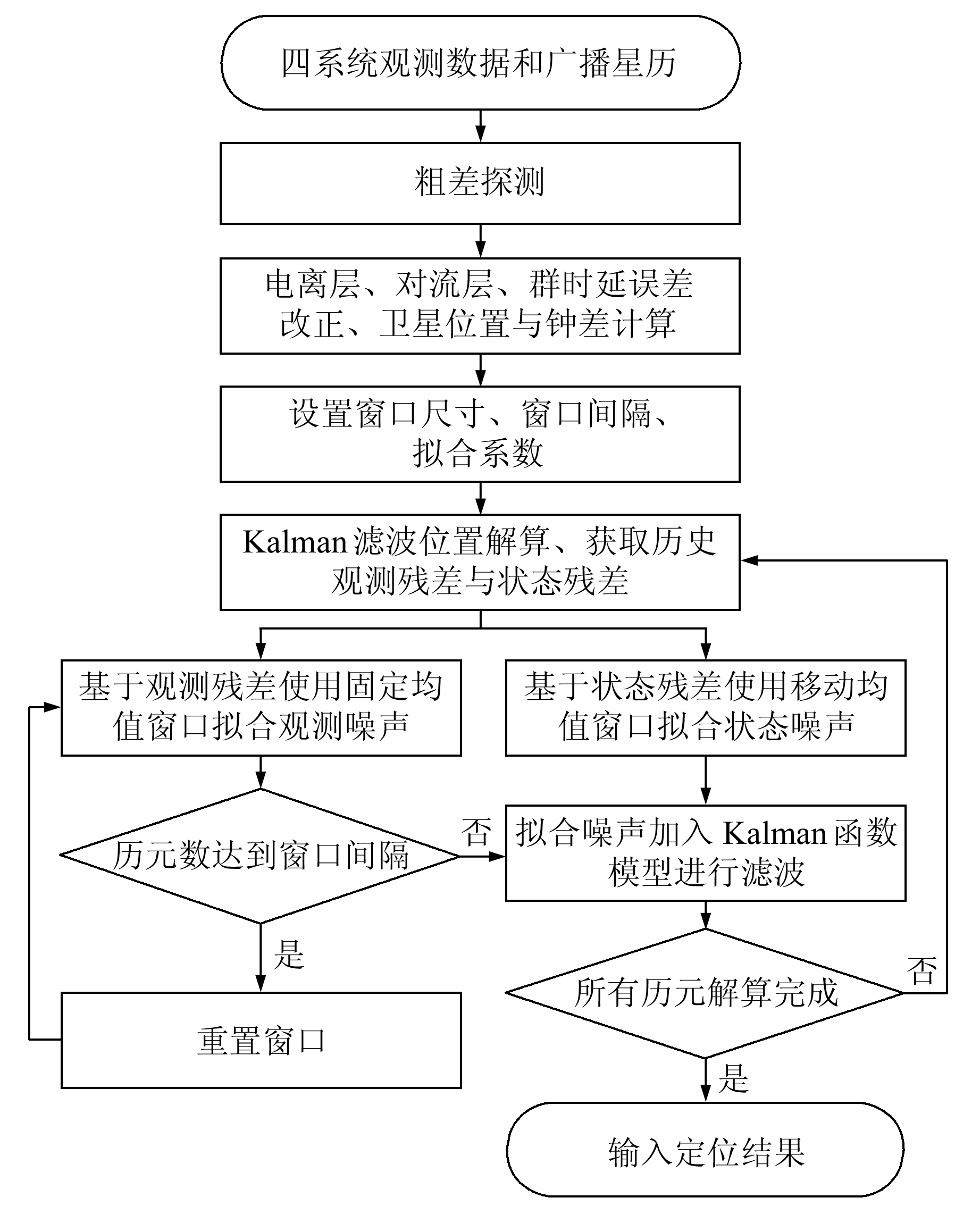图 1 基于有色噪声函数模型拟合的Kalman滤波位置解算算法流程 Fig. 1 Process of Kalman filtering algorithm for position calculating based on the color noise function model fitting
2 实验结果与分析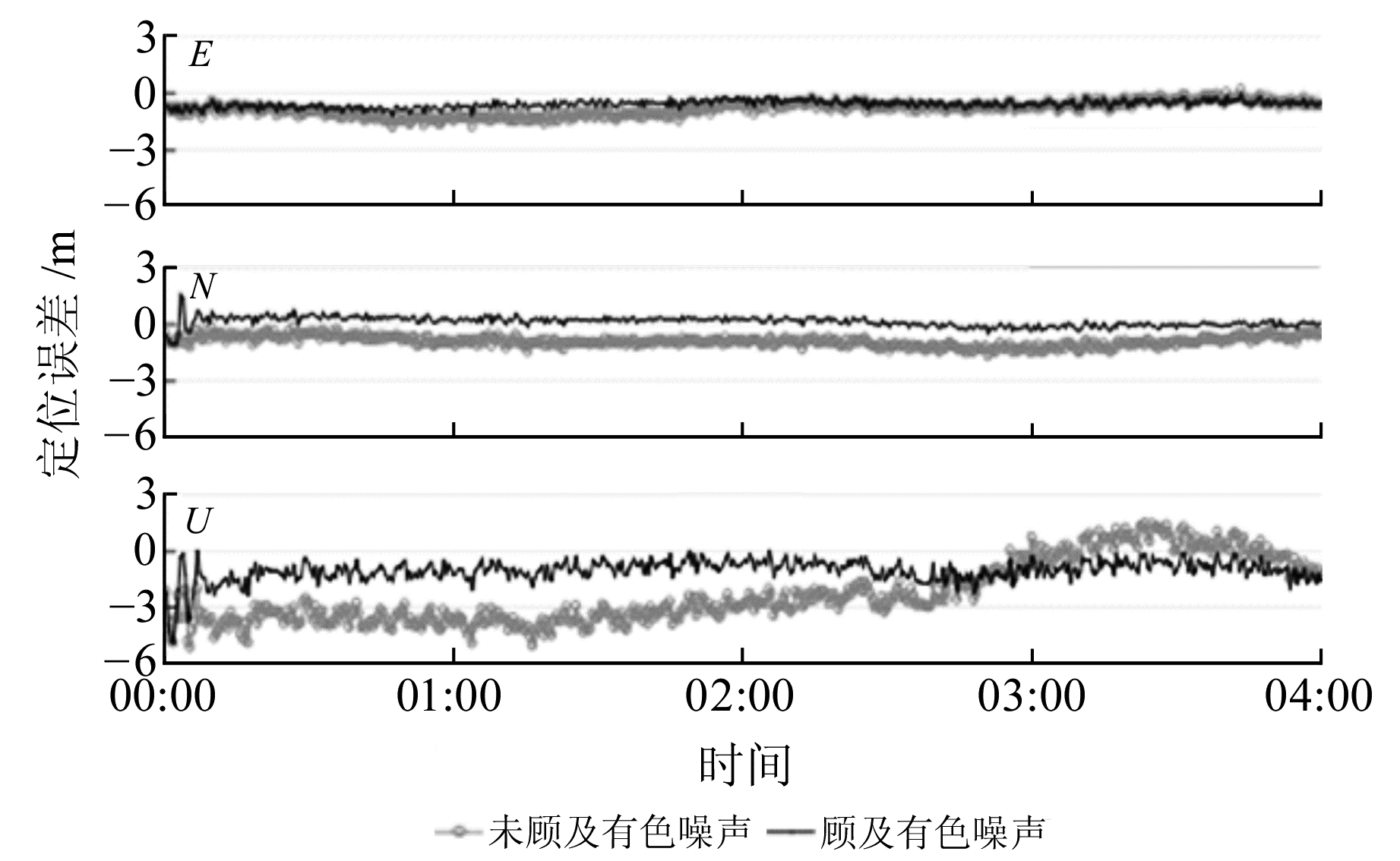图 2 KIRU测站3个方向定位误差对比 Fig. 2 Comparison of positioning errors in three directions at KIRU station表 1 KIRU测站单点定位精度统计 Tab. 1 Accuracy statistical table of single point positioning accuracy at KIRU station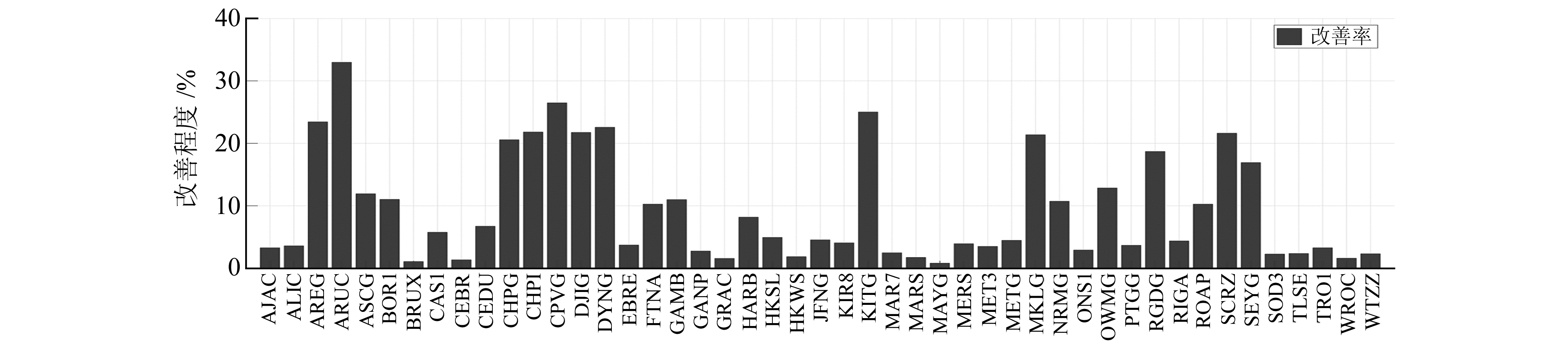图 3 使用函数模型拟合滤波算法的MGEX测站单点定位精度改善程度 Fig. 3 Improvement of accuracy of single point positioning accuracy at MGEX station by using function model fitting filtering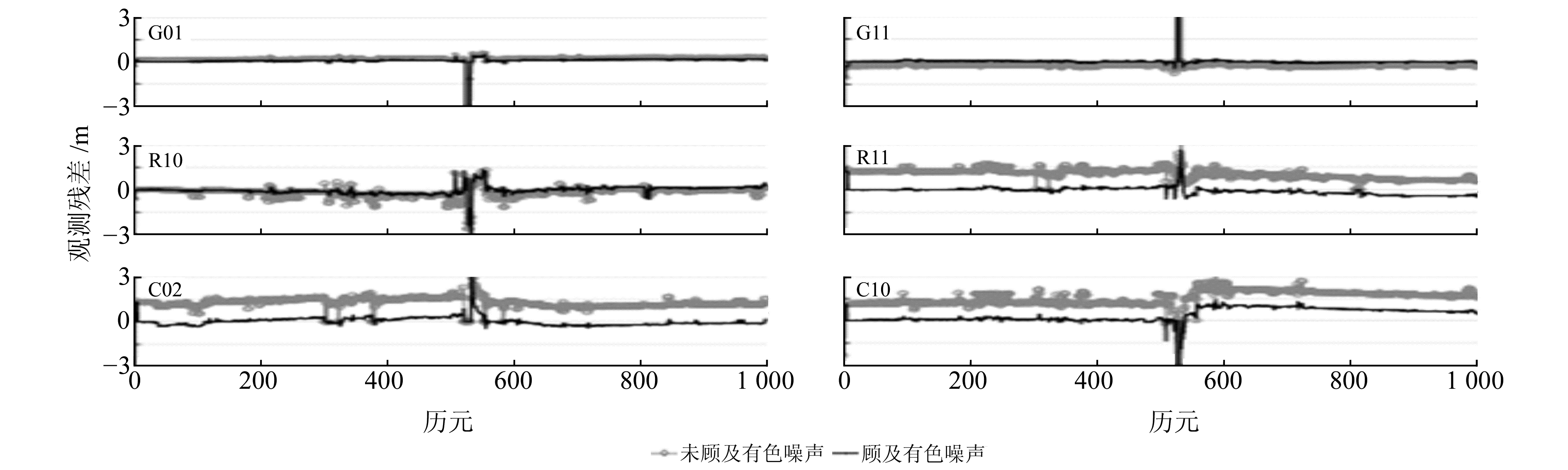图 4 动态定位中2颗不同GPS、GLONASS和BDS卫星的观测残差序列对比 Fig. 4 Comparison of observation residual sequences for two different GPS, GLONASS and BDS satellites kinematic positioning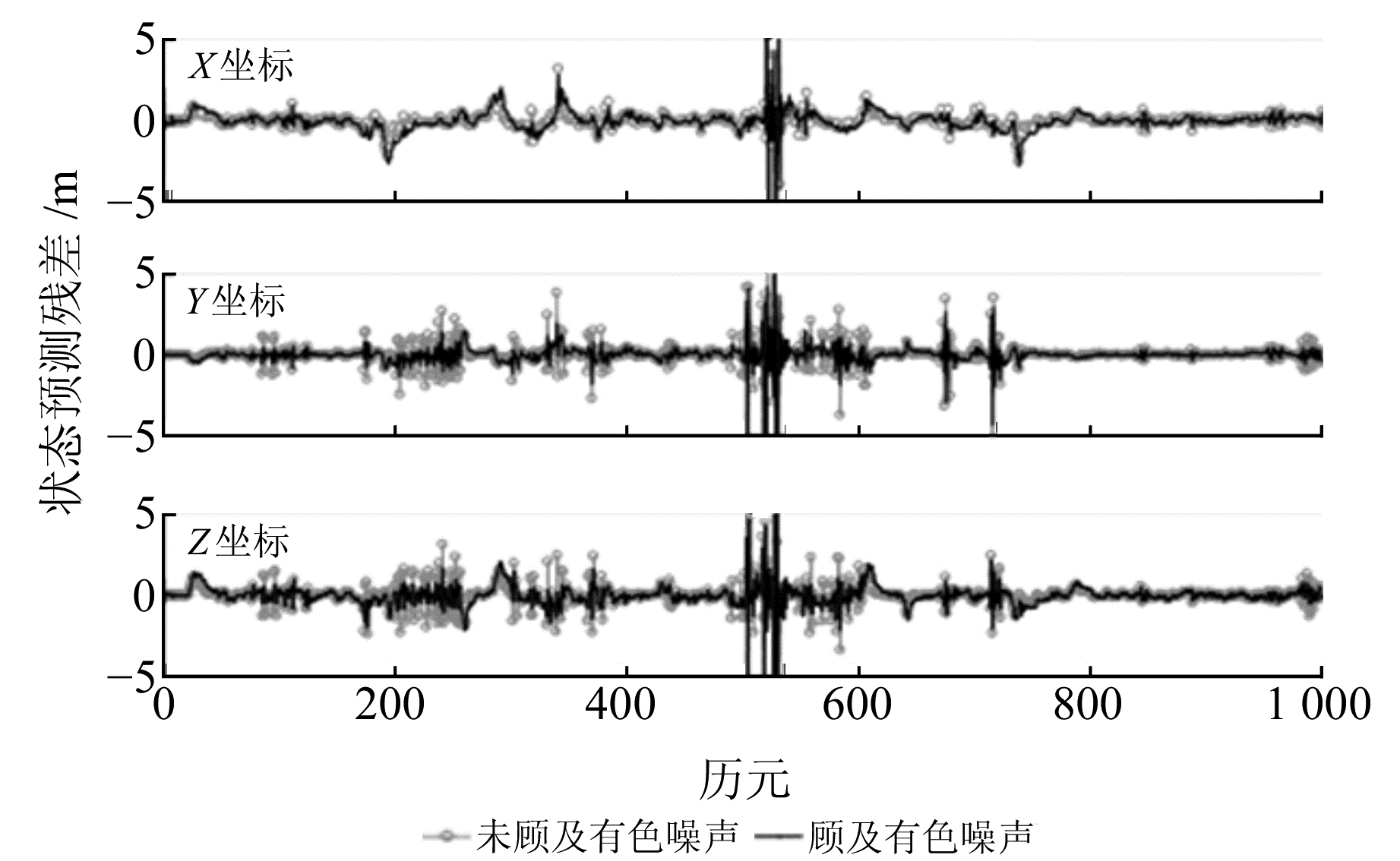图 5 动态定位状态预测残差序列对比表 2 动态单点定位精度统计 Tab. 2 Accuracy statistical of dynamic single point positioning
3 结语

  赵长胜, 陶本藻. 有色噪声作用下的卡尔曼滤波[J]. 武汉大学学报:信息科学版, 2008, 33(2): 180-182 (Zhao Changsheng, Tao Benzao. Kalman Filtering of Linear System with Colored Noises[J]. Geomatics and Information Science of Wuhan University, 2008, 33(2): 180-182) (0)  崔先强, 杨元喜, 高为广. 多种有色噪声自适应滤波算法的比较[J]. 武汉大学学报:信息科学版, 2006, 31(8): 731-735 (Cui Xianqiang, Yang Yuanxi, Gao Weiguang. Comparison of Adaptive Filter Arithmetics in Controlling Influence of Colored Noises[J]. Geomatics and Information Science of Wuhan University, 2006, 31(8): 731-735) (0)  Choi H D, Ahn C K, Lim M T. Time-Domain Filtering for Estimation of Linear Systems with Colored Noises Using Recent Finite Measurements[J]. Measurement, 2013, 46(1): 2792-2797 (0)  杨元喜, 任夏, 许艳. 自适应抗差滤波理论及应用的主要进展[J]. 导航定位学报, 2013, 1(1): 9-15 (Yang Yuanxi, Ren Xia, Xu Yan. Main Progress of Adaptively Robust Filter with Applications in Navigation[J]. Journal of Navigation and Positioning, 2013, 1(1): 9-15 DOI:10.3969/j.issn.2095-4999.2013.01.003) (0)  Sui L F, Mou Z K, Gan Y, et al. Unscented Kalman Filter Algorithm with Colored Noise and Its Application in Spacecraft Attitude Estimation[C]. The 1st International Workshop on the Quality of Geodetic Observation and Monitoring Systems, 2015 (0)  邹韬, 赵长胜, 丁圳祥. 有色观测噪声下的无迹卡尔曼滤波算法[J]. 测绘通报, 2015(6): 24-27 (Zou Tao, Zhao Changsheng, Ding Zhenxiang. Unscented Kalman Filter of Nonlinear Systems with Colored Measurement Noise[J]. Bulletin of Surveying and Mapping, 2015(6): 24-27) (0)  Cai C S, Gao Y, Pan L, et al. Precise Point Positioning with Quad-Constellations: GPS, Beidou, GLONASS and Galileo[J]. Advances in Space Research, 2015, 56(1): 133-143 DOI:10.1016/j.asr.2015.04.001 (0)  杨元喜. 自适应动态导航定位[M]. 北京: 测绘出版社, 2006 (Yang Yuanxi. Adaptive Navigation and Kinematic Positioning[M]. Beijing: Surveying and Mapping Press, 2006) (0)
A Filtering Algorithm for Quad-Constellation GNSS Kinematic Navigation with Consideration of Colored Noise
SUN Qingfeng1CAI Changsheng1     CUI Xianqiang1     YI Zhonghai1
1. School of Geosciences and Info-Physics, Central South University, 932 South-Lushan Road, Changsha 410083, China
Abstract: Classic Kalman filtering requires noise to be Gaussian white noise. However, the observation error and the state prediction error in GNSS kinematic positioning are colored noise. This paper establishes the colored noise model by using past observation residuals and state residuals in order to weaken the effects of colored noise on kinematic navigation solutions. Quad-constellations GNSS receiver measurements are used for a kinematic navigation experiment, and the results show that the algorithm can effectively improve positioning accuracy, as compared with the classic Kalman filtering algorithm with no consideration of the colored noise. The improvement rate of three-dimensional position accuracy is over 9%.
Key words: colored noise; kinematic navigation; filtering algorithm; GNSS